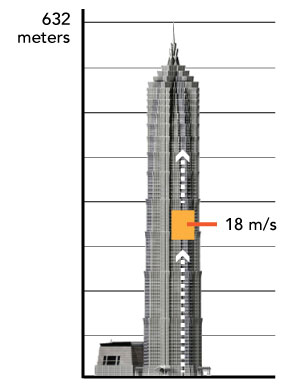Calculus

# Displacement, Velocity, Acceleration (Derivatives): Level 3 Challenges

The position of a particle is given by $p(t) = -t^2 + 4t$. Its journey starts at $t= 0$ and ends when it hits the $x$ axis again.

At what point in time $T$ will the particle have completed $75$% of its path (meaning 75% of the distance in the whole path is traveled by the particle)? Enter $\left \lfloor 1000 T \right \rfloor$ as your answer.The Shanghai Tower is the second tallest in the world, and has an architectural height of 632 meters. It is equipped with the world’s fastest elevator, which can travel at 18 m/s. Several newspapers reported that it will reach the top in just under 36 seconds, which isn't true due to physical limitations.

Firstly, the top floor of the building is only 557 meters high. Secondly, in order to prevent accidents to pregnant women, the maximum allowed acceleration is 1.25 m/s$^2$. Thirdly, for passenger comfort, the maximum jerk (rate of change of acceleration) is 2.50 m/s$^3$.

Based on these measurements, what is the shortest time that it would take an elevator to reach the top floor?

Details and assumptions

It actually takes the elevator 55 seconds to ascend 565 meters, due to further physical limitations. You can read more in the Mitsubishi Elevator Technical Paper.

The helicopter rises from rest on the ground vertically upwards with a constant acceleration $\dfrac g8$. A food packet is dropped from the helicopter when it has risen to a height $h$. What is the time taken by the food packet to reach the ground?A particle is executing 1D motion. Its acceleration as a function of time is given by $a=-Aw^{2}\sin(wt)$. At time $t$, the magnitude of displacement from the mean position, velocity, acceleration are equal. Then find the value of $t$.

Details and assumptions:

• At maximum acceleration(magnitude), velocity is 0
• At maximum velocity, displacement is zero
• $w$ is angular frequency
• 'A' a is constant of appropriate dimensionsWhen ships come in to dock where the berths are extremely narrow, the ships needs to be towed in to prevent damage to both the dock and the ship. A metal cable is attached to the front of the ship, looped through a pulley that is hinged at the edge of the dock, and attached to an automated winch.

At low tide, the top of the dock is 6 meters above sea level. The automated winch winds the cable at a constant rate of 10 meters per minute. To 2 decimal places, what is the speed (in meters per minute) at which the boat moves towards the shore when it is 10 meters from the shore?

×